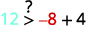# 3.5：绘制两个变量中的线性不等式

•• OpenStax
• OpenStax
$$\newcommand{\vecs}{\overset { \rightharpoonup} {\mathbf{#1}} }$$ $$\newcommand{\vecd}{\overset{-\!-\!\rightharpoonup}{\vphantom{a}\smash {#1}}}$$$$\newcommand{\id}{\mathrm{id}}$$ $$\newcommand{\Span}{\mathrm{span}}$$ $$\newcommand{\kernel}{\mathrm{null}\,}$$ $$\newcommand{\range}{\mathrm{range}\,}$$ $$\newcommand{\RealPart}{\mathrm{Re}}$$ $$\newcommand{\ImaginaryPart}{\mathrm{Im}}$$ $$\newcommand{\Argument}{\mathrm{Arg}}$$ $$\newcommand{\norm}{\| #1 \|}$$ $$\newcommand{\inner}{\langle #1, #2 \rangle}$$ $$\newcommand{\Span}{\mathrm{span}}$$ $$\newcommand{\id}{\mathrm{id}}$$ $$\newcommand{\Span}{\mathrm{span}}$$ $$\newcommand{\kernel}{\mathrm{null}\,}$$ $$\newcommand{\range}{\mathrm{range}\,}$$ $$\newcommand{\RealPart}{\mathrm{Re}}$$ $$\newcommand{\ImaginaryPart}{\mathrm{Im}}$$ $$\newcommand{\Argument}{\mathrm{Arg}}$$ $$\newcommand{\norm}{\| #1 \|}$$ $$\newcommand{\inner}{\langle #1, #2 \rangle}$$ $$\newcommand{\Span}{\mathrm{span}}$$$$\newcommand{\AA}{\unicode[.8,0]{x212B}}$$

##### 摘要

• 验证两个变量中不等式的解。
• 认识不等式解与其图之间的关系。
• 绘制两个变量中的线性不等式
• 使用两个变量中的线性不等式求解应用程序

1. 数字线$$x>2$$上的图形。
如果你错过了这个问题，请查看 [链接]
2. 解决：$$4x+3>23$$
如果你错过了这个问题，请查看 [链接]
3. 翻译：$$8<x>3$$
如果你错过了这个问题，请查看 [链接]

### 验证两个变量中不等式的解

##### 线性不等式

$$\begin{array} {l} { }& {Ax+By>C} &{Ax+By\geq C} &{Ax+By<C} &{Ax+By\leq C} \\ \end{array}$$图$$\PageIndex{1}$$

##### pageIndex {1}\)

ⓐ (0,0) (0,0) ⓑ (1,6) (1,6) ⓒ (2,6) (2,6) ⓓ (−5, −15) (−5, −15) ⓔ (−8,12) (−8,12)

 $$(0,0)$$简化。所以，$$(0,0)$$不是解决办法$$y>x+4$$。

 $$(1,6)$$简化。所以，$$(1,6)$$是一个解决方案$$y>x+4$$。

 $$(2,6)$$简化。所以，$$(2,6)$$不是解决办法$$y>x+4$$。

 $$(−5,−15)$$简化。所以，$$(−5,−15)$$不是解决办法$$y>x+4$$。

 $$(−8,12)$$简化。所以，$$(−8,12)$$是一个解决方案$$y>x+4$$。

$$(0,0)$$$$(4,9)$$$$(−2,1)$$$$(−5,−3)$$$$(5,1)$$

ⓐ 是的 ⓑ 是的 ⓒ 是的 ⓓ 是的 ⓔ 不是

##### 示例$$\PageIndex{3}$$

$$(0,0)$$$$(8,6)$$$$(−2,−1)$$$$(3,4)$$$$(−1,−4)$$

ⓐ 是的 ⓑ 是的 ⓒ 不 ⓓ 不 ⓔ 是的

## 认识不等式解与其图之间的关系图$$\PageIndex{2}$$：的解$$x>3$$是右边数字线的阴影部分$$x=3$$。

##### 边界线$\begin{array} {ll} {Ax+By<C} &{Ax+By\leq C} \\ {Ax+By>C} &{Ax+By\geq C} \\ {\text{Boundary line is }Ax+By=C.} &{\text{Boundary line is }Ax+By=C.} \\ {\text{Boundary line is not included in solution.}} &{\text{Boundary line is not included in solution.}} \\ {\textbf{Boundary line is dashed.}} &{\textbf{Boundary line is solid.}} \\ \nonumber \end{array}$图$$\PageIndex{3}$$

$\begin{array} {lll} {y} &{>} &{x+4} \\ {10} &{\overset{?}{>}} &{0+4} \\ {10} &{>} &{4} \\ \nonumber \end{array}$图$$\PageIndex{4}$$##### 示例$$\PageIndex{4}$$$$(0,0)$$，哪个不平等是正确的：还$$y>2x−1$$$$y<2x−1$$

$\begin{array} {ll} {y>2x−1} &{y<2x−1} \\ {0\overset{?}{>}2·0−1} &{0\overset{?}{<}2·0−1} \\ {0>−1\text{ True}} &{0<−1\text{ False}} \\ \nonumber \end{array}$

##### 示例$$\PageIndex{5}$$$$y\geq −2x+3$$

##### 示例$$\PageIndex{6}$$$$y\leq \frac{1}{2}x−4$$

##### 示例$$\PageIndex{7}$$$$(0,0)$$，哪个不平等是正确的：还$$2x+3y>6$$$$2x+3y<6$$

$\begin{array} {ll} {2x+3y>6} &{2x+3y<6} \\ {2(0)+3(0)\overset{?}{>}6} &{2(0)+3(0)\overset{?}{<}6} \\ {0>6\text{ False}} &{0<6\text{ True}} \\ \nonumber \end{array}$

（你可能想在边界线的另一边选一个点然后检查一下$$2x+3y>6$$。）

##### 示例$$\PageIndex{8}$$$$x−4y\leq 8$$

##### 示例$$\PageIndex{9}$$$$3x−y\geq 6$$

## 绘制两个变量中的线性不等式

##### 示例$$\PageIndex{10}$$: How to Graph a Linear Equation in Two Variables##### 示例$$\PageIndex{11}$$##### 示例$$\PageIndex{12}$$##### 绘制两个变量中的线性不等式。
1. 识别边界线并绘制图表。
• 如果不等式为\ leq 或\ geq、\ leq 或\ geq，则边界线为实线。
• 如果不等式为 <or><or>，则边界线为虚线。
2. 测试不在边界线上的点。 这是不平等的解决方案吗？
3. 边界线一侧的阴影。
• 如果测试点是解，则在包含该点的一侧加上阴影。
• 如果测试点不是解决方案，则在另一侧遮阳。
##### 示例$$\PageIndex{13}$$$$(0,0)$$有解决方案$$x−2y<5$$吗？##### 示例$$\PageIndex{14}$$##### 示例$$\PageIndex{15}$$##### 示例$$\PageIndex{16}$$$$(1,0)$$有解决方案$$y\leq −4x$$吗？##### 示例$$\PageIndex{17}$$##### 示例$$\PageIndex{18}$$$\begin{array} {ll} {x=a} &{\text{vertical line}} \\ {y=b} &{\text{horizontal line}} \\ \nonumber \end{array}$

##### 示例$$\PageIndex{19}$$

$y>3\nonumber$$0\slashed{>}3\nonumber$##### 示例$$\PageIndex{20}$$##### 示例$$\PageIndex{21}$$## 使用两个变量中的线性不等式求解应用程序

##### 示例$$\PageIndex{22}$$

ⓐ 让 xx 成为她在餐饮服务部门工作的小时数，让 y 成为她工作辅导的小时数。 写一个不等式来模拟这种情况。

ⓑ 绘制不等式图。

ⓒ 找到三个可以解决$$(x,y)$$不等式的有序对。 然后，解释这对希拉里亚意味着什么。

ⓐ 我们让 x 成为她在餐饮服务部门工作的小时数，让 y 成为她工作辅导的小时数。ⓑ 为了绘制不等式图，我们将其绘制为斜率截距形式。

\begin{align} {10x+15y} &\geq 240 \\ 15y &\geq -10x+240 \\ y &\geq {−\frac{2}{3}x+16} \\ \nonumber \end{align}ⓒ 从图中我们可以看出，有序对$$(15,10)$$$$(24,0)$$代表无限多解中的三个。$$(0,16)$$ 检查不等式中的值。##### 示例$$\PageIndex{23}$$

Hugh 有两份兼职工作。 一个在杂货店，每小时支付10美元，另一个是保姆，每小时13美元。 在这两份工作之间，休希望每周至少赚260美元。 Hugh 每份工作需要工作多少小时才能赚到至少 260 美元？

ⓐ 假设 x 是他在杂货店工作的小时数，让 y 成为他保姆的小时数。 写一个不等式来模拟这种情况。

ⓑ 绘制不等式图。

ⓒ 找到三个可以解决不等式的有序对（xy）。 然后，解释一下这对休意味着什么。

$$10x+13y\geq 260$$ⓒ 答案会有所不同。

##### 示例$$\PageIndex{24}$$

ⓐ 让 x 成为她在日间水疗中心工作的小时数，让 y 成为她担任行政助理的小时数。 写一个不等式来模拟这种情况。

ⓑ 绘制不等式图。

ⓒ 找到三个可以解决不等式的有序对（xy）。 然后，解释一下这对维罗妮卡意味着什么

$$10x+17.5y\geq 280$$ⓒ 答案会有所不同。

## 关键概念

• 如何在两个变量中绘制线性不等式。
1. 识别边界线并绘制图表。
如果不等式为$$\leq$$$$\geq$$，则边界线为实线。
如果不等式为$$<$$$$>$$，则边界线为虚线。
2. 测试不在边界线上的点。 这是不平等的解决方案吗？
3. 边界线一侧的阴影。
如果测试点是解，则在包含该点的一侧加上阴影。
如果测试点不是解决方案，则在另一侧遮阳。